Next: Stokes' flow Up: Fluid flow at low Previous: Fluid flow at low

## Poiseuille flow

We'll start with the flow of a viscous fluid in a channel. The channel has a width in the y-direction of a, a length in the z-direction of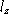, and a length in the x-direction, the direction of flow, of . There is a pressure drop along the length of the channel, so that the constant pressure gradient is (such a pressure gradient could be supplied by gravity, for instance). Assuming the flow to be steady,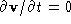. Also, we'll assume that the flow is of the form ; then . The no-slip boundary condition at the top and bottom edges of the channel reads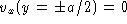. The Navier-Stokes equation then becomes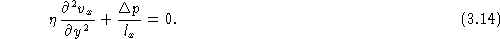Integrating twice, we obtain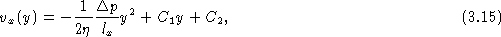where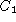and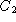are integration constants. To determine these, we impose the boundary conditions to obtainWe see that the velocity profile is a parabola, with the fluid in the center of the channel having the greatest speed. Once we know the velocity profile we can determine the flow rate Q, defined as the volume of fluid which passes a cross section of the channel per unit time. This is obtained by integrating the velocity profile over the cross sectional area of the channel: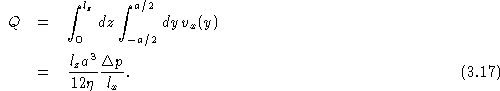The analogous result for flow through a pipe of radius a and length l in the presence of a uniform pressure gradient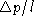is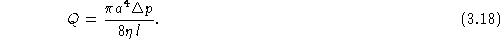The important feature of both of these results is the sensitive dependence upon either the channel width a or the pipe radius a. For instance, for a pipe with a fixed pressure gradient, a 20% reduction in the pipe radius leads to a 60% reduction of the flow rate! This clearly has important physiological implications -- small amounts of plaque accumulation in arteries can lead to very large reductions in the rate of blood flow.Next: Stokes' flow Up: Fluid flow at low Previous: Fluid flow at low

Vittorio Celli
Sun Sep 28 22:13:11 EDT 1997